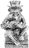### Database table not saved? NX script of a single if statement works and produces a view that look correct. But click off to another table and then return to the table and it is empty. How can this be?

NX Script

delete (select SquareHLTPs);
for n in select 'Cycle Times' do
let j := (create SquareHLTPs);
j.(TP_StartDate := n.Start_Date);
j.(Top_Yes := n.Start_Type);
j.(TP_Price := n.Start_TP_Price);
j.('8x1_Date' := n.Start_Date + n.Start_TP_Price * 12.5);
j.('4x1_Date' := n.Start_Date + n.Start_TP_Price * 25);
j.('3x1_Date' := n.Start_Date + n.Start_TP_Price * 33.3334);
j.('2x1_Date' := n.Start_Date + n.Start_TP_Price * 50);
j.('1x1_Date' := n.Start_Date + n.Start_TP_Price * 100);
j.('1x2_Date' := n.Start_Date + n.Start_TP_Price * 200);
j.('1x3_Date' := n.Start_Date + n.Start_TP_Price * 333.4);
j.('1x4_Date' := n.Start_Date + n.Start_TP_Price * 400);
j.('1x8_Date' := n.Start_Date + n.Start_TP_Price * 800)
end

Issue is that it works fine after 20 mins of processing to produce 16000 records in the view on screen but doesnt appear to save it.

I click onto another table check it and then go back to the first table only to find that it is empty.

How can this be?You are declaring variable j each time your for-loop iterates. You declare a variable once and assign values to it as many times as you like. Try this...

`delete (select SquareHLTPs);`

`let j := (create SquareHLTPs);`
`for n in select 'Cycle Times' do`
`j.(TP_StartDate := n.Start_Date);`
`j.(Top_Yes := n.Start_Type);`
`j.(TP_Price := n.Start_TP_Price);`
`j.('8x1_Date' := n.Start_Date + n.Start_TP_Price * 12.5);`
`j.('4x1_Date' := n.Start_Date + n.Start_TP_Price * 25);`
`j.('3x1_Date' := n.Start_Date + n.Start_TP_Price * 33.3334);`
`j.('2x1_Date' := n.Start_Date + n.Start_TP_Price * 50);`
`j.('1x1_Date' := n.Start_Date + n.Start_TP_Price * 100);`
`j.('1x2_Date' := n.Start_Date + n.Start_TP_Price * 200);`
`j.('1x3_Date' := n.Start_Date + n.Start_TP_Price * 333.4);`
`j.('1x4_Date' := n.Start_Date + n.Start_TP_Price * 400);`
`j.('1x8_Date' := n.Start_Date + n.Start_TP_Price * 800);`

`j := (create SquareHLTPs);`
`end`

I think this will leave you with an empty record though.

Thanks I had looked at this for a couple of hours and couldn’t see it.